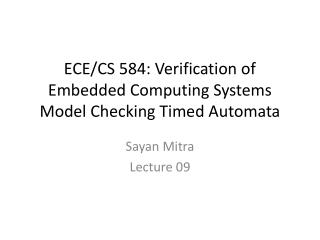# ECE/CS 584: Verification of Embedded Computing Systems Model Checking Timed Automata - PowerPoint PPT PresentationDownload PresentationECE/CS 584: Verification of Embedded Computing Systems Model Checking Timed Automata

ECE/CS 584: Verification of Embedded Computing Systems Model Checking Timed AutomataDownload Presentation## ECE/CS 584: Verification of Embedded Computing Systems Model Checking Timed Automata

- - - - - - - - - - - - - - - - - - - - - - - - - - - E N D - - - - - - - - - - - - - - - - - - - - - - - - - - -
##### Presentation Transcript

1. ECE/CS 584: Verification of Embedded Computing SystemsModel Checking Timed Automata SayanMitra Lecture 09

2. What we have seen so far • A very general modeling framework (Lynch et al.’s Hybrid Automata) • Complex discrete dynamics • Possibly nonlinear continuous dynamics • Distributed • General proof techniques for the above model • Inductive invariants for proving safety • Simulation relations for trace inclusion • Introduction to a General-purpose theorem prover (PVS) and examples of mechanizing proofs for state machines • How to model state machines in PVS • How to construct invariant proofs • Can be partially automated but requires a lot of manual work  Nancy Lynch, Roberto Segala, and Frits Vaandrager. Hybrid I/O automata. Information and Computation, 185(1):105-157, August 2003.

3. Next • Focus on specific classes of Hybrid Automata for which safety properties (invariants) can be verified completely automatically • Alur-Dill’s Timed Automata (Today) • Rectangular initializaed hybrid automata • Linear hybrid automata • … • Later we will look at other types of properties like stability, liveness, etc. • Abstractions and invariance are still going to be important  Rajeev Alur et al. The Algorithmic Analysis ofHybrid Systems. Theoretical Computer Science, colume 138, pages 3-34, 1995.

4. Today • Algorithmic analysis of (Alur-Dill’s) Timed Automata • A restricted class of what we call hybrid automata in this course with only clock variables  Rajeev Alur and David L. Dill. A theory of timed automata. Theoretical Computer Science, 126:183-235, 1994.

5. Clocks and Clock Constraints • A clock variable x is a continuous (analog) variable of type real such that along any trajectory of x, for all t . • For a set X of clock variables, the set (X) of integral clock constraints are expressions defined by the syntax: g ::= x where • Examples: x = 10; x [2, 5); true are valid clock constraints • Semantics of clock constraints

6. Integral Timed Automata • Definition. A integral timed automaton is a HIOA A = where • V = X , where is a set of n clocks and is a discrete state variable of finite type Ł • A is a finite set • is a set of transitions such that • The guards are described by clock constraings • implies either or • set of clock trajectories for the clock variables in X

7. Example: Light switch Description Switch can be turned on whenever at least 2 time units have elapsed since the last turn off. Switches off automatically 15 time units after the last on. Math Formulation automaton Switch variables internal x, y:Real := 0, loc: {on,off} := off transitions internal push pre x 2 effif loc= on then y := 0 fi; x := 0; loc:= off internal pop pre y = 15 /\ loc= off effx := 0 trajectories invariant loc = on \/ loc = off stop when y = 15 /\ loc = off evolved(x) = 1; d(y) = 1

8. Control State (Location) Reachability Problem • Given an ITA, check if a particular location is reachable from the initial states • This problem is decidable • Key idea: • Construct a Finite State Machine that is a time-abstract bisimilar to the ITA • Check reachability of FSM

9. A Simulation Relation with a finite quotient • When two states x1 and x2 in Q behave identically? • x1.and • x1 and x2 satisfy the same set of clock constraints • For each clock int(x1.)=int(x2.)or int(x1.and int(x2.. ( of ) • For each clock with x1., frac(x1.ifffrac(x2. • For any two clocks and with x1. and x1., frac(x1.)frac(x1.) ifffrac(x2.) frac(x2.) • Lemma. This is a equivalence relation on Q • The partition of Q induced by this relation is are called clock regions

10. What do the clock regions look like? Example of Two Clocks X = {y,z} = 2 = 3

11. Complexity • Lemma. The number of clock regions is bounded by |X|! 2|X|.

12. Region Automaton • ITA (clock constants) defines the clock regions • Now we add the “appropriate transitions” between the regions to create a finite automaton which gives a time abstract bisimulationof the ITA with respect to control state reachability • Time successors: Consider two clock regions , we say that is a time successor of if there exits a trajectory of ITA starting from that ends in ’ • Discrete transitions: Same as the ITA

13. Time Successors The clock regions in blue are time successors of the clock region in red.

14. Example 1: Region Automata ITA Corresponding FA

15. Example 2 ITA Clock Regions

16. Corresponding FA |X|! 2|X| Drastically increasing with the number of clocks

17. Summary • ITA: (very) Restricted class of hybrid automata • Clocks, integer constraints • No clock comparison, linear • Control state reachability • Alur-Dill’s algorithm • Construct finite bisimulation (region automaton) • Idea is to lump together states that behave similarly and reduce the size of the model • UPPAAL model checker based on similar model of timed automata